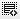## Salary expenses - Quiz Question0
In the Dominos Pizza Income Statement (from the video), the Salary expense grows by how much % from 2008 to 20090

The income statement can be found on page 13 of this document - http://www.sebi.gov.in/dp/jubilantdraft.pdf1

if we look in to the income statement of Dominos, we can find the following salary expense figures of 2008 and 2009

2008 the salary expenses was - 425.07
2009 the salary expense was - 555.67

the increase in the salary expenses from 2008 to 2009 is 555.67- 425.07 = 130.6

If the same has to be shown in percentage, the calculation would be as under

for 425.07 the net increase is 130.6
for 100 (since we want the answer in %) - how much is the increase?

425.07 -------------- 130.6
100 ------------------ ?

100/ 425.07 * 130.6 = 30.72 or 0.31% (approx)

Click on thisicon to add code snippet.